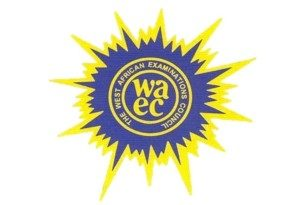## WAEC 2019 CHEMISTRY PRACTICAL VERIFIED EXPO ANSWERS(1a)

DRAW A TABLE WITH THE FOLLOWING

Final |13.60|15.50|14.80|12.50

Initial |1.00 |3.10 |2.40 |0.00

Vol. acid used |12.60|12.60|12.40|12.50

Volume of S2O3²- =12.60+12.40+12.50/3

=37.50/3

=12.50cm³

(1bi)

Conc. in g/dm³ = molar mass * conc in mol/dm³

But molar mass of Na2S2O3

=(23*2)+(32*2)+(16*3)

=46 + 64 + 48

=158g/mol

Therefore 15.8g/dm³ = 158g/mol * conc of A in mol/dm³

Conc of A in mol/dm³ = 15.8/158

=0.1mol/dm³

(1bii)

Using CaVa/CbVb = na/nb

0.1*12.50/Cb * 25.00 = 2/1

50Cb = 1.25

Cb = 1.25/50

Cb = 0.025mol/dm³

The conc of I2 in B = 0.025mol/dm³

(1biii)

Gram conc of I2 = molar mass * molar conc

= (127*2)*0.025

=6.35g/dm³

Percentage mass of I2 in sample = 6.35/9.0 *100%

= 0.7056 * 100%

=70.56%

(1c)

There have to be a change in the colour of the mixture before it is added. The end point is known when the blue colour formed as starch is added, changes to colourless

=======================

(3ai)

On adding BaCl2 solutions to a portion of saturated Na2CO3 precipitate is formed,precipitate dissolves on adding excess dilute HCl

(3aii)

Q is reducing agent like SO2, H2S, CO

(3bi)

calcium oxide(Cao)

(3bii)

concentrated H2SO4

(3c)

NaOH pellet is delinquescent because it absorb moisture from the atmosphere to form solution

========================

(2a)
Test: C + Water and filtered

Observation: part of C dissolves, colour less filtrate is formed

Inference: C is a mixture of a soluble salt

(2bi)
Test: 2cm^2 of filterate of salt C + AgNO3(aq) then dilute HNO3

Observation: white precipitate is formed

Inference: Cl^- present

(2bii)
Test: Solution im 2(b)(i) + excess NH3 solution

Observation: precipitate disorder in excess solution of NH3

Inference; Cl^- confirmed

(2ci)
Test: Resident + dilute HCl + shake

Observation; residue dissolved to form a blue solution

CHECK OUT:  WAEC 2019 LITERATURE IN ENGLISH DRAMA AND POETRY VERIFIED ANSWER

Inference; Cu^2+ present

(2cii)
Test; solution in 2(c)(i) + NH3 solution in drops and then in excess

Observation: light pale blue precipitate is formed precipitate dissolved in excess solution of NH3 to give a deep blue solution

Inference; Cu^2+ confirmed.Join Our Telegram Channel |Whatsapp Our Admins: Click Any Name Mr.Paul or Mr.Henry

### Comment

Posted by on 3rd May 2019.

Categories: WAEC